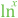# LN10

 Block Group: Constants Icon:The LN10 block is a Number block. When the LN10 block is created, the LN10 block holds the mathematical constant for the natural logarithm of ten.

For information on using dataflow blocks, see Dataflow.

## Input/Output Property

The following property of the LN10 block can take input and give output.

• value (number)

value sets and returns the number held by this LN10 block. When the LN10 block is created, the value property holds the mathematical constant for the natural logarithm of ten.

## Example

The following image demonstrates an example of the LN10 block.